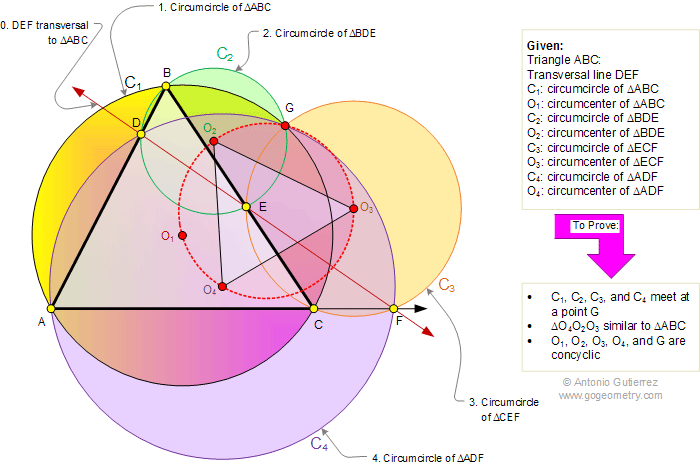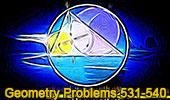# Problem 548: Triangle, Transversal, Complete Quadrilateral, Circumcircles, Circumcenters, Similarity, Concyclic Points. Level: High School, SAT Prep, College Geometry

 The figure shows a triangle ABC with a transversal DEF (F on the extension of AC). O1, O2, O3, and O4 are the circumcenters of triangles ABC, BDE, ECF, and ADF, respectively. Prove that (1) the circumcircles of triangles ABC, BDE, ECF, and ADF meet at a point G, (2) Triangles ABC and O4O2O3. are similar, (3) O1, O2, O3, O4, and G are concyclic points (lie on a same circle). Post a comment or solution.More Geometry Problems:Problem 547. Triangle, Transversal, Four Circumcircles, Concurrency. Problem 546. Triangle, Angle Trisectors, 60 Degrees, Equilateral triangle. Problem 545. Acute Triangle, Squares, Altitudes, Area, Measure.
 Home | Search | Geometry | Problems | All Problems | Visual Index | 541-550 | Email | By Antonio Gutierrez